# Gimbal derotated video, using clouds as the horizon

#### Mick West

Staff member
Here's az = 45° with the above correction.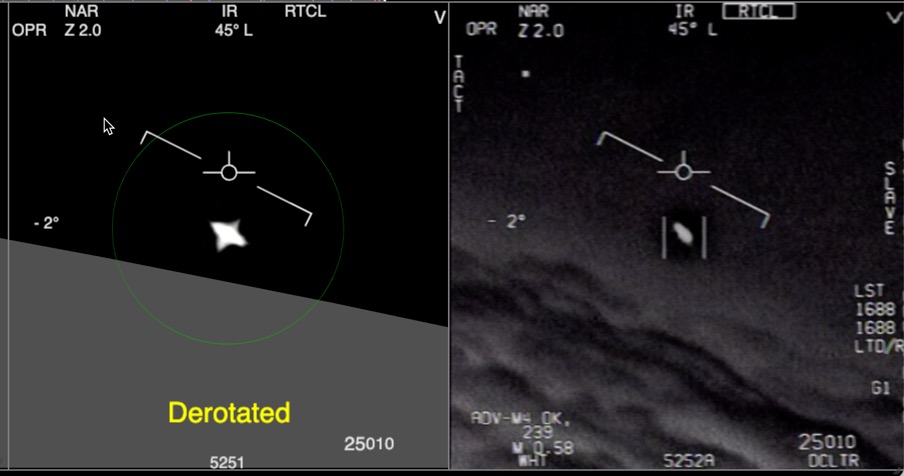None of this changes the first observable - there are still significant shifts in the horizon angle due to jet roll that are not reflected in the glare rotation.

It does introduce a little motion in the glare, with might explain the (so far debatable) appearance of slight CW rotation in the glare in the video over the first 20 seconds.

Dinner time.

#### logicbear

##### New Member
It does introduce a little motion in the glare
Is it supposed to ? No matter how the jet rotates relative to the horizon, the angle of the glare should be unaffected as long as the derotation device doesn't kick in ? Is the change showing some additional pod roll ?

#### Mick West

Staff member
Is it supposed to ? No matter how the jet rotates relative to the horizon, the angle of the glare should be unaffected as long as the derotation device doesn't kick in ? Is the change showing some additional pod roll ?
The derotation has to derotate to a specific angle. If there's bank and/or pitch then this changes as the azimuth changes

#### dimebag2

##### Active Member
This discussion has been very helpful in understanding the artificial/real horizon mismatch (at least for me). My take on all this as of now :

1) the whole image is expected to rotate CW, not only during banking episodes, but also from a gradual realignment of the image with horizon indicator as the Az gets closer to 0 (the cos(Az) term)

2) the close flight path predicts a slow CCW rotation, with perspective changes as it tilts upwards (assuming the object rotates along the path). The glare hypothesis predicts no rotation other than pod roll, because the glare must be fixed in the camera.

If we loop frames 11 and 372 (beginning/end of white hot segment), we get this :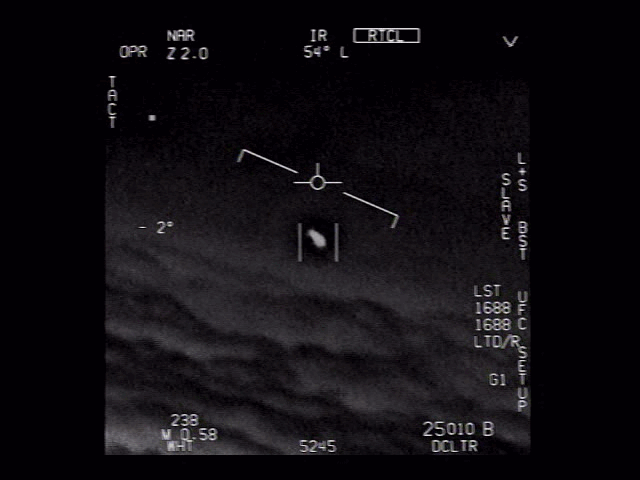Defining the object's axis is ambiguous, but I think there is a discernible CW rotation here (but less than the clouds). Two options :
- it's a glare, and this means the pod has had a bit of CW rotation
- it's a physical object, it rotated CCW along flight path, but just a bit less than the CW rotation of the image. In other words the two rotations counteracted each other, but realignment of the image "won" by a bit.

Now, let's compare the black hot segment, before any "step" rotation (frames 401-720):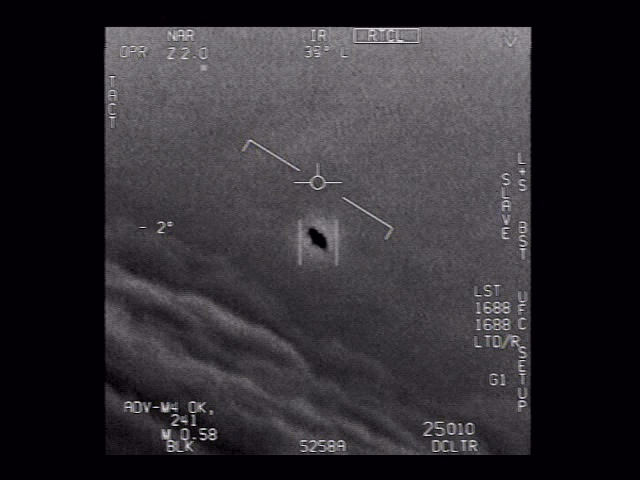Again, the shape changes so its not clear if the object's axis is exactly fixed or not. As before, there may be a bit of CW rotation here (look at the axis of the little spikes on each side), that could be due to slight CW pod roll if a glare, or cancellation of CCW object's rotation along flight path versus CW realignment of the image, as before.

My take on this :

- The 1st observable is ambiguous, because what we see is not incompatible with how a real physical object would look throughout the vid, accounting for cancellation between predicted CCW rotation of the object, and CW realignment of the image.

- A glare fits what we see, at least for the "fixed in camera frame part", provided that there is a bit of CW rotation in pod roll over the first 24s.

Now I agree this would be a strange coincidence that the object rotates CCW while the image realigns CW, making it appear fixed in the image.
But the close flight path predicts a tilt of ~10-15° CCW over the first 24s (see below, it starts aligned with the blue line), which is not far from the difference of rotation between the clouds and the object (clouds tilt by ~18°).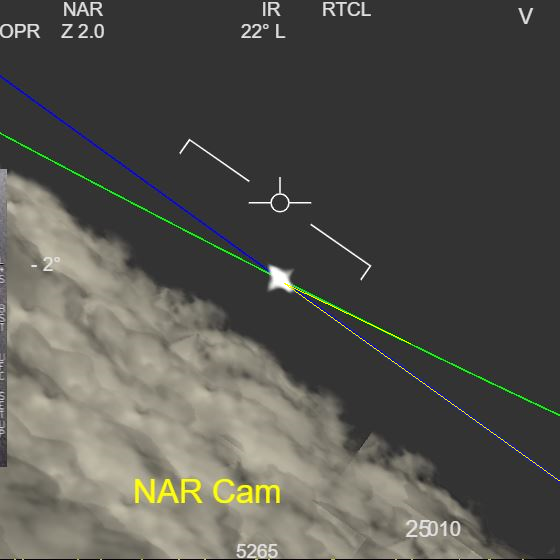As you know I favor the close flight path to the distant plane scenario, because it explains what the aviators report. I'm not discussing if it is likely or not, I simply want to verify if what we see in the vid may fit this scenario. Like I said before it does for flight path and SA stop/reverse, and accounting for CCW rotation of the object along flight path, it kinda does too. This can be another coincidence and another "trick of the glare". Or the object is in fact rotating in the scene. I keep an open mind.

To go further on this, I have asked Mick to add a 3D flying saucer model in Sitrec, as he did with a F-18 for FLIR1. Why not, after all? This way we can check if the shape we see at the end (saucer-like), would match the shape we see earlier, based on what change in perspective along the close flight path is predicted by the 3D recreation. If it's not a physical object, but a glare, there should be no match, or this would be another weird coincidence.

A loose estimation of how a physical object would rotate along close flight path, if it tilts by 10-20° :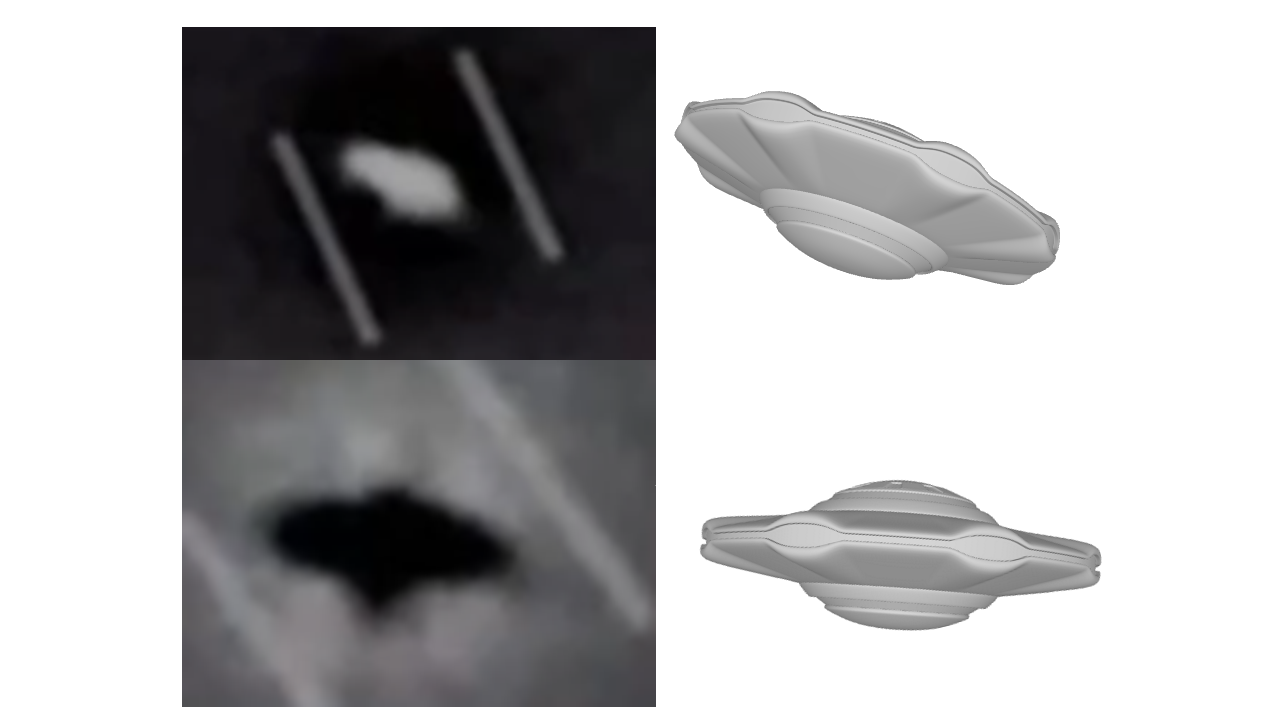It'd be cool to check this on Sitrec, with precise changes in distance/angle of view included.
Thanks Mick for agreeing to do it, I think it's a fun thing to look at.

#### Mick West

Staff member
- The 1st observable is ambiguous, because what we see is not incompatible with how a real physical object would look throughout the vid, accounting for cancellation between predicted CCW rotation of the object, and CW realignment of the image.

- A glare fits what we see, at least for the "fixed in camera frame part", provided that there is a bit of CW rotation in pod roll over the first 24s.

Now I agree this would be a strange coincidence that the object rotates CCW while the image realigns CW, making it appear fixed in the image.
That "strange coincidence" is the first observable. Of course, you could posit that it's a flying saucer that just happens to rotate (or rotate a lot more) at the same time the jet banks.

I eventually did some of the math to get the horizon angle correction for looking sideways. The problem is that it comes out twice as big as the observed angle change of the clouds. I did it three different ways and got the same result. A simple way to fix it was to half the result.

JavaScript:
``````function getDesiredHorizonAngleFrame(frame) {

var jetPitch = jetPitchFromFrame(frame) // this will get scaled pitch
var jetRoll = jetRollFromFrame(frame)
var az = Frame2Az(frame)

// What angle should the horizon be at in the ATFLIR
// if looking straight ahead it's par.jetRoll
// if looking left or right it's par.jetPitch
// to find the horizon angle we take the up vector and rotate it by roll and pitch

var jetUp = new THREE.Vector3(0, 1, 0)

jetUp.applyAxisAngle(V3(0, 0, 1), -radians(jetRoll))

// // to apply pitch, we need to first calculate the rolled right axis
var rightAxis = V3(1,0,0)
//  rightAxis.applyAxisAngle(V3(0, 0, 1), -radians(jetRoll))

// // now we can rotate in local space.

// then rotate by -az to make the view plane lay along the Z axis
jetUp.applyAxisAngle(V3(0, 1, 0), -radians(az))

// then the angle is just from x and y
var desiredAngle =  degrees(Math.atan2(jetUp.x, jetUp.y))

// ad hoc scaling of difference from jetRoll to match observed cloud motion
desiredAngle = 0.5 * (desiredAngle - jetRoll) + jetRoll

return desiredAngle
}``````

This suggest to me that there's some human factors consideration in calculating the derotation correction when looking sideways. i.e. it's not reducing the roll angle (of the image in the ATFLIR) as much as it mathematically should do - instead it's reducing it in a way that feels good to the pilot.

Or I could be missing something. But the end result has to match the cloud motion.

The end result is a pretty small shift in the initial "ideal" camera roll. I need more time to really nail it down though.

Last edited:

#### jarlrmai

##### Senior Member
That "strange coincidence" is the first observable. Of course, you could posit that it's a flying saucer that just happens to rotate (or rotate a lot more) at the same time the jet banks.

I eventually did some of the math to get the horizon angle correction for looking sideways. The problem is that it comes out twice as big as the observed angle change of the clouds. I did it three different ways and got the same result. A simple way to fix it was to half the result.

JavaScript:
``````...
}``````

This suggest to me that there's some human factors consideration in calculating the derotation correction when looking sideways. i.e. it's not reducing the roll angle (of the image in the ATFLIR) as much as it mathematically should do - instead it's reducing it in a way that feels good to the pilot.

Or I could be missing something. But the end result has to match the cloud motion.

The end result is a pretty small shift in the initial "ideal" camera roll. I need more time to really nail it down though.
So there internally there is some algorithm that adjusts the de-rotation ammount based on the horizontal angle of the ATFLIR look direction? So there are probably more variables that are there based on adjustment to what "felt right" when testing.

Last edited by a moderator:

#### dimebag2

##### Active Member
That "strange coincidence" is the first observable. Of course, you could posit that it's a flying saucer that just happens to rotate (or rotate a lot more) at the same time the jet banks.

I would agree with this if the image (the clouds) was closely rotating with sudden changes in banking. But it's not the case over the first 24s. See below this graph by logicbear. Only at 20s we more clearly see an impact of banking in the clouds angle. Which makes sense as banking gets clearer in the horizon line with Az decreasing : Pitch*sin(Az)->0 and Bank*cos(Az)->Bank.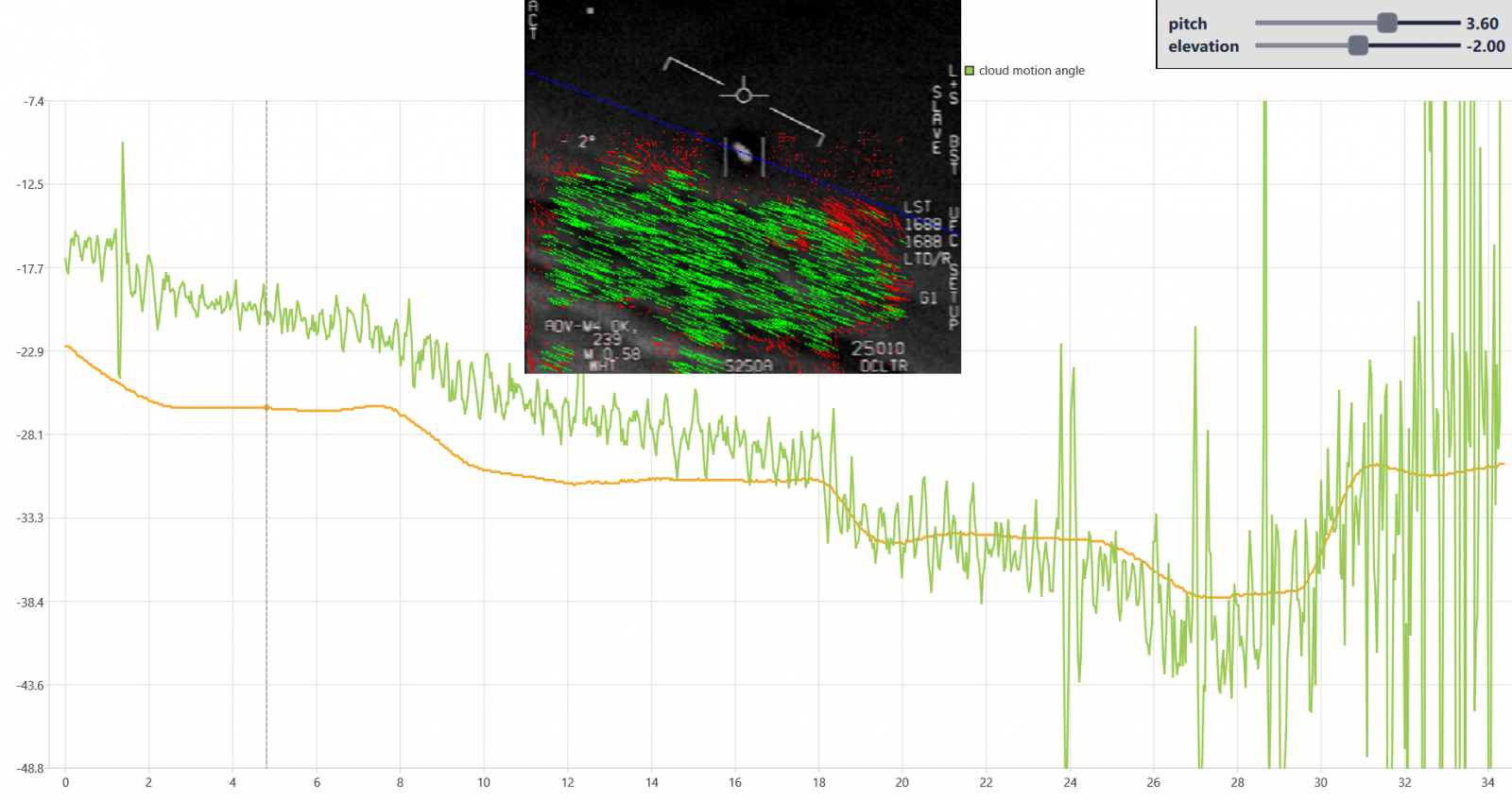Applying a 10deg CCW correction to the object angle (linear here, it should be curved as tilt rate increases with time), we get this comparison between cloud angles and object's angle, over the first 24s.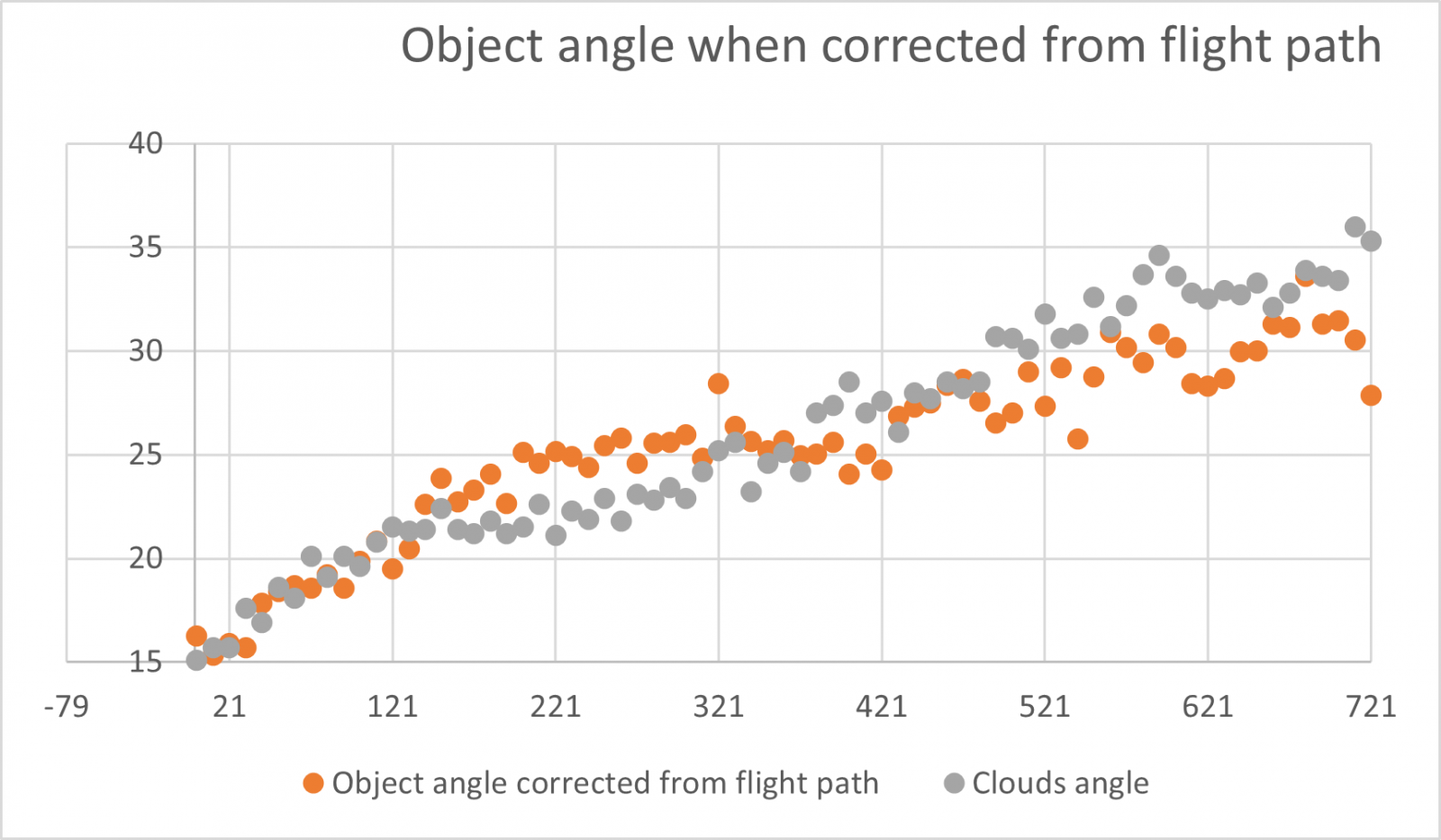The changes in banking are not extremely clear in the clouds, and given the uncertainty when defining the object's angle, it's not way off.

My point is, if it was a physical object tilting along the close flight path, it would not deviate from the clouds much during banking, in those first 24s. If it's a glare, it's not obvious it's fixed in the camera frame during sudden changes in banking, because the image itself (the clouds) does not closely follow them (except at 20s, which was shown earlier in this thread).

Last edited:

#### dimebag2

##### Active Member
This suggest to me that there's some human factors consideration in calculating the derotation correction when looking sideways. i.e. it's not reducing the roll angle (of the image in the ATFLIR) as much as it mathematically should do - instead it's reducing it in a way that feels good to the pilot.

Or I could be missing something. But the end result has to match the cloud motion.

The end result is a pretty small shift in the initial "ideal" camera roll. I need more time to really nail it down though.

Could pitch explain the difference here? What pitch do you need to explain the difference in predicted clouds angle, versus what we observe?

jetPitch = (ObservedCloudAngle - (jetRoll*cos(abs(radians(az)))) / sin(abs(radians(az)))

Also in this equation jetPitch is relative to elevation of the pod right? (-2°)

Last edited:

#### Mick West

Staff member
I would agree with this if the image (the clouds) was closely rotating with sudden changes in banking. But it's not the case over the first 24s. See below this graph by logicbear. Only at 20s we more clearly see an impact of banking in the clouds angle. Which makes sense as banking gets clearer in the horizon line with Az decreasing : Pitch*sin(Az)->0 and Bank*cos(Az)->Bank.
It certainly diminishes in significance with the greater Az, but it's still there. You can see the slope change in the graph.
My point is, if it was a physical object tilting along the close flight path, it would not deviate from the clouds much during banking, in those first 24s. If it's a glare, it's not obvious it's fixed in the camera frame during sudden changes in banking, because the image itself (the clouds) does not closely follow them (except at 20s, which was shown earlier in this thread).
Coding it as if it's a glare gives essentially this graph (but using the 0.5x scale) There are no points at which it deviates.

#### Mick West

Staff member
Could pitch explain the difference here? What pitch do you need to explain the difference in predicted clouds angle, versus what we observe?
You'd need a negative pitch, which isn't really possible as a constant (discussed earlier.)

Also in this equation jetPitch is relative to elevation of the pod right? (-2°)
No, pitch, is the angle of the jet's axis relative to the ground plane. It's an aerodynamic thing. The pod elevation is just where the camera is looking.

The "Observered Cloud Angle" should be the angle of the horizon on the ATFLIR, which is jet roll + pod roll - derotation.

Jet roll is the bank angle as indicated on screen.

I'd previously had derotation = pod roll (which was a slight simplification, even after ignoring the side view correction).

Now we have
JavaScript:
``````var horizonAngle = getDesiredHorizonAngleFrame(par.frame)
var podHorizonAngle = getPodHorizonAngleFrame(par.frame)
var deroNeeded = podHorizonAngle - horizonAngle``````

getDesiredHorizonAngleFrame is described above.

getPodHorizonAngleFrame is
JavaScript:
``````// to get the frame of reference of the pod camera, we can just extract it from the ball's matrixWorld
// We duplicate that object hierarchy here so we can extract the code

function getPodHorizonAngleFrame(frame) {
// similar to UpdatePRFromEA, but on locals, not par values
var pitch, roll;
var az = Frame2Az(frame)
var el = par.el; // TODO - in sitrec this can change per frame
[pitch, roll] = EAJP2PR(el, az, jetPitchFromFrame(frame));
var podPitchPhysical = pitch;
var globalRoll = roll
var jetRoll = jetRollFromFrame(frame)
var podRollIdeal = globalRoll-jetRoll;
var podRollPhysical = podRollIdeal
if (par.podRollFromGlare) {
podRollPhysical = GlareAngleFromFrame(frame)
}

var jetPitch = jetPitchFromFrame(frame)
var jetRoll = jetRollFromFrame(frame)

var localBall = new THREE.Object3D()
var localEOSU = new THREE.Object3D()
var localPodFrame = new THREE.Object3D()
localPodFrame.updateMatrixWorld()
localEOSU.updateMatrixWorld()
localBall.updateMatrixWorld()

var podHorizonAngle = degrees(extractRollFromMatrix(localBall.matrixWorld))

return podHorizonAngle;
}

// given a rotation matrix m, it's comprised of orthogonal x,y, and z basis vectors
// which define an object or camera orientation
// -z is the forward basis, meaning that it's the direction the camera is looking in
// x and y are rotated around the z-axis by the roll angle
// the roll angle is the angle or y from a vector orthogonal to z and pointing up
// find the angle the y basis vector is rotated around the z basis vector
// from a y-up orientation
function extractRollFromMatrix(m) {
var xBasis = V3();
var yBasis = V3();
var zBasis = V3();
m.extractBasis(xBasis, yBasis, zBasis)
xBasis.normalize()
yBasis.normalize()
zBasis.normalize()

// right is ortogonal to the forward vector and the global up
var right = zBasis.clone().cross(V3(0,1,0))

// yUP is the y basis roated upright
var yUp = right.clone().cross(zBasis)

// so calculate how much we rotated it
var angle = yUp.angleTo(yBasis)

// flip depending on which side of the plane defined by the right vector
if (right.dot(yBasis) > 0 )
angle = -angle;

return angle
}``````

Which is a lot of code to answer the question, "what's the angle of the horizon looking through the pod right now?" The end result does not differ hugely from the pod roll, but I want to be as thorough as possible.

#### logicbear

##### New Member
I eventually did some of the math to get the horizon angle correction for looking sideways. The problem is that it comes out twice as big as the observed angle change of the clouds. I did it three different ways and got the same result. A simple way to fix it was to half the result.
I have a version (green line) that gets really close to the interpolated values (blue line) without the 0.5 scale issue. Am I missing something ?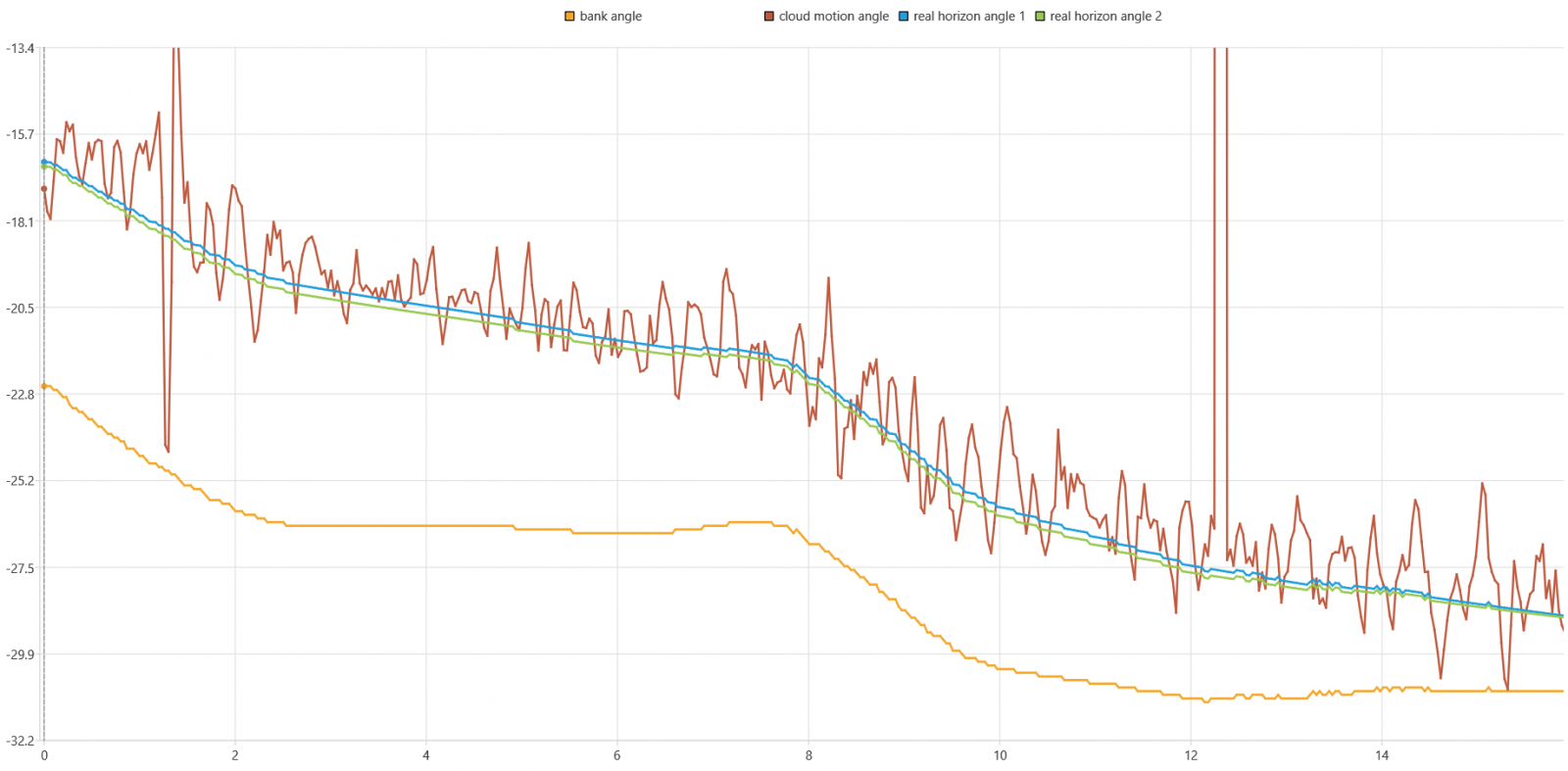I'm assuming that it's intuitive for pilots to look left/right, up/down, but not to tilt their head, so I try to recreate what the horizon would look like if you had a camera strapped to the jet that can only rotate left/right in the wing plane, or up/down perpendicular to that. First I find how much I need to rotate the camera along those lines to look directly at the object, then I compute the angle between a vector pointing right in the camera plane, and the real horizon given by a vector that is tangential to the global 'az' viewing angle.
C++:
``````double get_real_horizon_angle_for_frame(int frame, int type = 2) {
double el = Frame2El(frame), az = Frame2Az(frame);
double jetPitch = jetPitchFromFrame(frame), jetRoll = jetRollFromFrame(frame);

if (type == 1) {
return jetRoll * cos(radians(az)) + jetPitch * sin(radians(az));
} else {
// rotate the absolute 3D coordinates of (el, az) into the frame of reference of the jet
vec3d relative_AzElHeading = EA2XYZ(el, az, 1)
.rotate(vec3d { 1, 0, 0 }, -radians(jetPitch)) // reverse both the order and sign of these rotations
.rotate(vec3d { 0, 0, 1 }, radians(jetRoll));
// caclulcate (el, az) angles relative to the frame of reference of the jet
auto [relative_el, relative_az] = XYZ2EA(relative_AzElHeading);

// compute the jet's pose in the global frame of reference
auto jetUp = vec3d { 0, 1, 0 }
.rotate(vec3d { 0, 0, 1 }, -radians(jetRoll))
.rotate(vec3d { 1, 0, 0 }, radians(jetPitch));
auto jetRight = vec3d { 1, 0, 0 }
.rotate(vec3d { 0, 0, 1 }, -radians(jetRoll))
.rotate(vec3d { 1, 0, 0 }, radians(jetPitch));

// rotate the camera by relative_az in the wing plane so that it's looking at the object
// the camera pitching up by relative_el has no effect on a vector pointing right
auto camera_horizon = jetRight.rotate(jetUp, -radians(relative_az));

// the real horizon is a vector pointing right, perpendicular to the global viewing angle az
auto real_horizon = vec3d { 1, 0, 0 }.rotate(vec3d { 0, 1, 0 }, -radians(az));

// it can be shown that the real horizon vector is already in the camera plane
// so return the angle between the camera horizon and the real horizon
return -degrees(camera_horizon.angleTo(real_horizon));
}
}``````

Last edited:

#### dimebag2

##### Active Member
I did my own extraction of cloud angles/banking (every 10 frames), I get this plot when comparing Clouds angle and predicted clouds angle, with jet pitch=3.6 (from the horizon formula). Its close to the graph of logicbear above (I have my angles positive versus negative, just a display thing).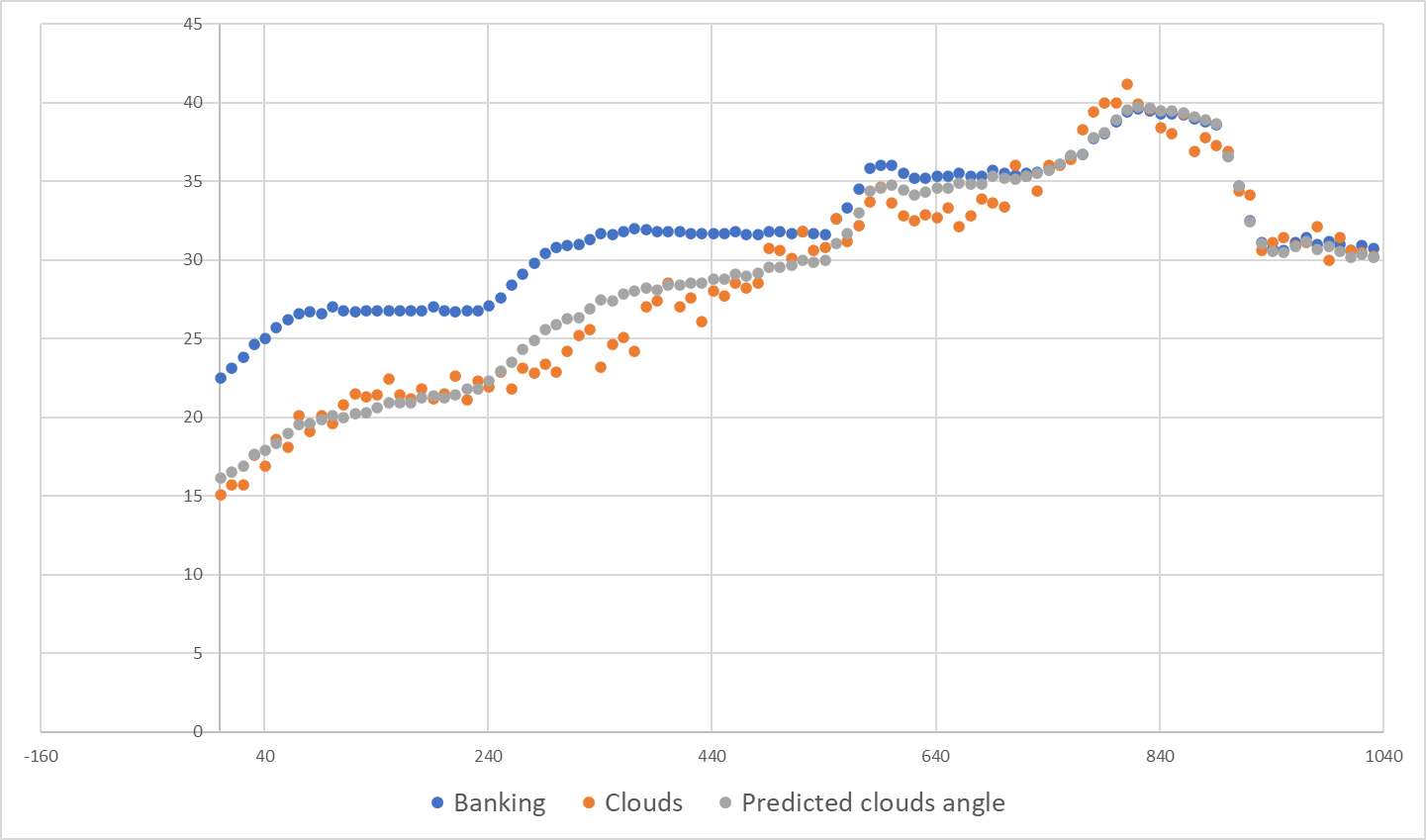Estimating what the pitch should be at any given time, to match the observed clouds angle, I get this (with shifted banking for display) :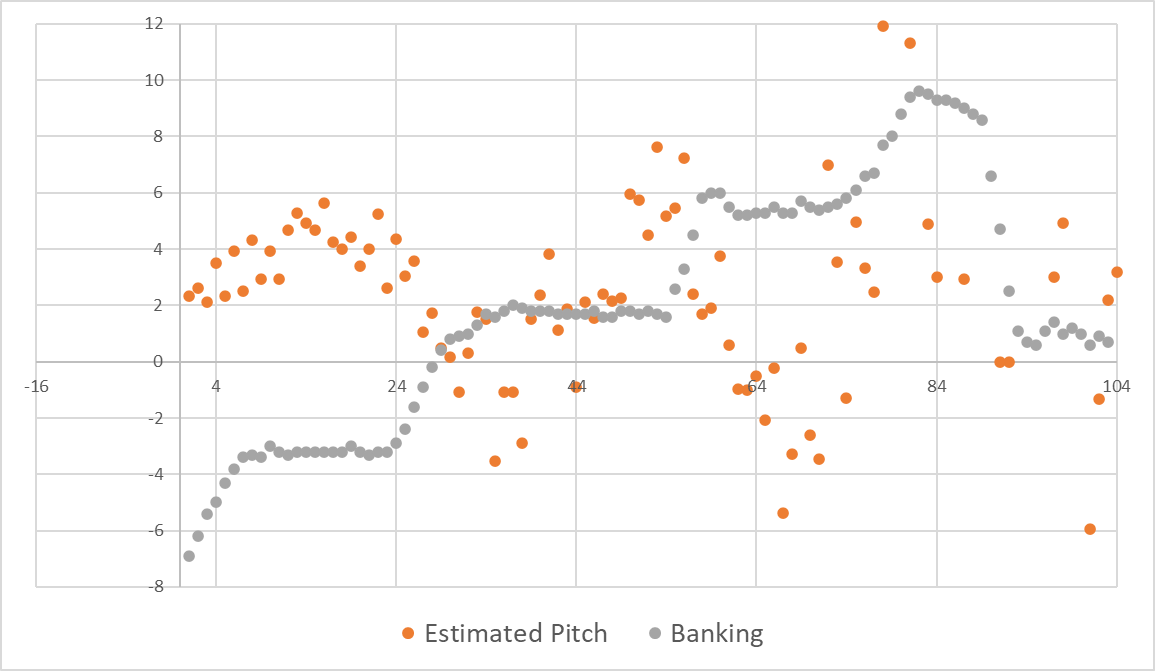There may be significant short variations in pitch during flight? Note as Az decreases the effect of pitch gets indiscernible and it gets all very noisy.

Last edited:

#### Mick West

Staff member
I have a version (green line) that gets really close to the interpolated values (blue line) without the 0.5 scale issue. Am I missing something ?
Probably not. I replicated your code in Javascript and got the same result as you. I think it's valid. I've got some other work I need to do, and I want to make sure I fully understand what is going on. But I'll try to update both sims early next week.

JavaScript:
``````// https://www.metabunk.org/threads/gimbal-derotated-video-using-clouds-as-the-horizon.12552/page-2#post-276183
//double get_real_horizon_angle_for_frame(int frame, int type = 2) {
function get_real_horizon_angle_for_frame(frame) {
//    double el = Frame2El(frame), az = Frame2Az(frame);
//    double jetPitch = jetPitchFromFrame(frame), jetRoll = jetRollFromFrame(frame);

var jetPitch = jetPitchFromFrame(frame) // this will get scaled pitch
var jetRoll = jetRollFromFrame(frame)
var az = Frame2Az(frame)
var el = par.el;

//     if (type == 1) {
//         return jetRoll * cos(radians(az)) + jetPitch * sin(radians(az));
//     } else {
//         // rotate the absolute 3D coordinates of (el, az) into the frame of reference of the jet
//         vec3d relative_AzElHeading = EA2XYZ(el, az, 1)
//             .rotate(vec3d { 1, 0, 0 }, -radians(jetPitch)) // reverse both the order and sign of these rotations
//              .rotate(vec3d { 0, 0, 1 }, radians(jetRoll));

var relative_AzElHeading = EA2XYZ(el, az, 1)
.applyAxisAngle(V3(1, 0, 0), -radians(jetPitch))
.applyAxisAngle(V3(0, 0, 1), radians(jetRoll))

//         // caclulcate (el, az) angles relative to the frame of reference of the jet
//         auto [relative_el, relative_az] = XYZ2EA(relative_AzElHeading);
var relative_el, relative_az;
[relative_el, relative_az] = XYZ2EA(relative_AzElHeading)

//
//         // compute the jet's pose in the global frame of reference
//         auto jetUp = vec3d { 0, 1, 0 }
//     .rotate(vec3d { 0, 0, 1 }, -radians(jetRoll))
//     .rotate(vec3d { 1, 0, 0 }, radians(jetPitch));
var jetUp = V3(0, 1, 0)
.applyAxisAngle(V3(0, 0, 1), -radians(jetRoll))
.applyAxisAngle(V3(1, 0, 0), radians(jetPitch))

//         auto jetRight = vec3d { 1, 0, 0 }
//     .rotate(vec3d { 0, 0, 1 }, -radians(jetRoll))
//     .rotate(vec3d { 1, 0, 0 }, radians(jetPitch));
var jetRight = V3(1, 0, 0)
.applyAxisAngle(V3(0, 0, 1), -radians(jetRoll))
.applyAxisAngle(V3(1, 0, 0), radians(jetPitch))

DebugArrowV("jetUp",jetUp)

//         // rotate the camera by relative_az in the wing plane so that it's looking at the object
//         // the camera pitching up by relative_el has no effect on a vector pointing right
//         auto camera_horizon = jetRight.rotate(jetUp, -radians(relative_az));
var camera_horizon = jetRight.applyAxisAngle(jetUp, -radians(relative_az));

DebugArrowV("camera_horizon",camera_horizon,100,0xff0000) // red

//         // the real horizon is a vector pointing right, perpendicular to the global viewing angle az
//         auto real_horizon = vec3d { 1, 0, 0 }.rotate(vec3d { 0, 1, 0 }, -radians(az));
var real_horizon = V3(1, 0, 0).applyAxisAngle(V3(0, 1, 0), -radians(az))
DebugArrowV("real_horizon",real_horizon,100,0x00ff00) // green

//
//         // it can be shown that the real horizon vector is already in the camera plane
//         // so return the angle between the camera horizon and the real horizon
//         return -degrees(camera_horizon.angleTo(real_horizon));
return -degrees(camera_horizon.angleTo(real_horizon));
//     }
// }
}``````

(That's from the Gimbal sim, so a fixed el)

#### logicbear

##### New Member
Here's a shorter, simpler version of the same. I suspect the other one might work better when the pod is looking straight down, but for our purposes the results are identical. This one should produce a horizon angle with the opposite sign when the jet is banking the other way.
C++:
``````// Compute the jet's pose in the global frame of reference.
auto jetUp = vec3d { 0, 1, 0 }
.rotate(vec3d { 0, 0, 1 }, -radians(jetRoll))
.rotate(vec3d { 1, 0, 0 }, radians(jetPitch));

// Get a vector pointing at the object in the global frame of reference.
auto AzElHeading = EA2XYZ(el, az, 1);

// First the camera is rotated left/right in the wing plane, around the up axis,
// so that forward, up and AzElHeading are all in the same plane. At this point
// a vector pointing right, the camera_horizon, is perpendicular to AzElHeading and jetUp.
// Rotating the camera up/down around camera_horizon has no effect on camera_horizon.
auto camera_horizon = AzElHeading.cross(jetUp); // 0 if the pod is looking straight down

// The real horizon is a vector pointing right, perpendicular to the global viewing angle az.
auto real_horizon = vec3d { 1, 0, 0 }.rotate(vec3d { 0, 1, 0 }, -radians(az));

// Both camera_horizon and real_horizon are perpendicular to AzElHeading
// so they are already in the same plane, the camera plane,
// so return the signed angle between them using https://stackoverflow.com/a/33920320
double dot = camera_horizon.dot(real_horizon);
double cross_dot_n = camera_horizon.cross(real_horizon).dot(AzElHeading);
return -degrees(atan2(cross_dot_n, dot));``````

Last edited: